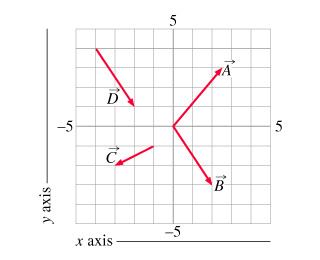# Problem: The following questions will ask you to give both components of vectors using the ordered pairs method. In this method, the x component is written first, followed by a comma, and then the y component. For example, the components of A_vec would be written 2.5,3 in ordered pair notation.The answers below are all integers, so estimate the components to the nearest whole number.What is true about B_vec and D_vec? Choose from the pulldown list below.a. They have different components and are not the same vectors.b. They have the same components but are not the same vectors.c. They are the same vectors.

###### FREE Expert Solution

Bx, By = 2,-3

Dx, Dy = 2,-3###### Problem Details

The following questions will ask you to give both components of vectors using the ordered pairs method. In this method, the x component is written first, followed by a comma, and then the y component. For example, the components of A_vec would be written 2.5,3 in ordered pair notation.

The answers below are all integers, so estimate the components to the nearest whole number.

What is true about B_vec and D_vec? Choose from the pulldown list below.

a. They have different components and are not the same vectors.

b. They have the same components but are not the same vectors.

c. They are the same vectors.